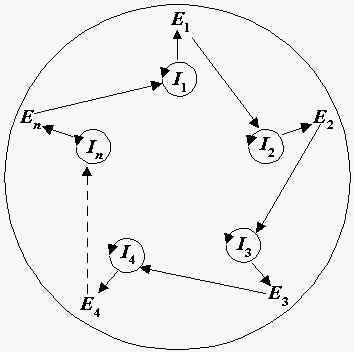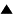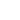# Hypercycles

M. Eigen and P. Schuster proposed the model of hypercycles , as a hypothetical stage of macromolecular evolution, which could follow quasispecies.

The hypercycle is a self-reproducing macromolecular system, in which RNAs and enzymes cooperate in the following manner (Fig.1): there are RNA matrices ( Ii ); i-th RNA codes i-th enzyme Ei (i = 1,2,...,n); the enzymes cyclically increase RNA's replication rates, namely, E1 increases replication rate of I2 , E2 increases replication rate of I3 , ..., En increases replication rate of I1 . In addition, the mentioned macromolecules cooperate to provide primitive translation abilities, so the information, coded in RNA-sequences, is translated into enzymes, analogously to the usual translation processes in biological objects. The cyclic organization of the hypercycle ensures its structure stability. For effective competition, the different hypercycles should be placed in separate compartments.Fig. 1. Hypercycle structure. Ii are RNA matrices, Ei are replication enzymes (i = 1,2,...,n).

The replication enzymes ensure the more accurate RNAs' replication as compared with quasispecies, providing opportunities for further macromolecular structure improvements. M. Eigen and P. Schuster consider hypercycles as predecessors of protocells (primitive unicellular biological organisms) .

Let's review shortly the mathematical description of hypercycles, taking into account the original model  as well as the analysis by R.Feistel, Yu. M. Romanovskii, and V.A.Vasil'ev , who modeled a hypercycles competition in coacervate-type compartments.

We suppose, that 1) the hypercycles are placed into coacervates ; 2) each coacervate includes only one type of the hypercycles; 3) any coacervate volume is proportional to the number of macromolecules inside it; 4) a translation process is much quicker than a replication one (the latter means that it is sufficient to consider the RNAs dynamics only ). Using these assumptions, we obtain the following equations:
 dNi /dt = Vfi , V = c-1 Si Ni , xi = Ni /V , i = 1,2,..., n, (1)

where Ni and xi are a number of molecules of i-th RNA and its concentration in a coacervate, respectively; V is a coacervate volume; c is a constant, characterizing a total concentration of macromolecules in a given coacervate; fi is a synthesis rate of i-th RNA. Values fi are defined as:
 fi = a xi xj , i = 1,2,..., n, j = i -1 + ndi1, (2)

where a is a synthesis rate parameter (for the sake of simplicity we assume the value a to be the same for all RNAs in a given hypercycle), dij is the Kronecker symbol (dij = 1, if j = i; dij = 0, otherwise). Eq. (2) takes into account the cyclic structure of hypercycles (see Fig.1). From (1) we obtain:
 dxi /dt = fi - xi c-1 Sj fj , (3)
 dV/dt = V c-1 Sj fj . (4)

According to (2),(3), the concentration dynamics in a particular hypercycle is described by the nonlinear ordinary differential equations, which were analyzed  by qualitative methods. The analysis showed, that if the number of hypercycle member n is less than or equal to 4, the macromolecules concentrations xi converge to the following equilibrium values:
 x0i = c /n, (5a)

and if n > 4 the concentrations xi converge to a stable limit cycle, that is a periodic orbit in the concentration hyperspace. For the limit cycles, the mean values of the macromolecules concentrations xi are determined by the same formula  :
 = c /n. (5b)

If several hypercycles have different replication abilities, their volumes increase with different rates. Averaging on periodic concentration oscillations (if needed), we can deduce, that according to (4), the hypercycle, having the greatest value
 Wk = < ck-1 Sj fkj > (6)

(k is the hypercycle type index), possesses the greatest selective ability.

In order to consider the hypercycles competition explicitly, let's suppose, that any coacervate splits into two parts, when its volume exceeds a certain critical value, and that the total volume of all types of hypercycles is restricted by some constant: Sk Vk = VT = const (Vk is the total volume of k-th type of hypercycles). Then instead of Eq. (4), we have:
 dVk/dt = Wk Vk - Vk [VT-1SkWkVk] . (7)

The selective values Wk can be calculated from (2), (3), (5), (6) :
 Wk = ak ck /nk (8)

Eq. (7) is well known ; according to its solution, only the hypercycle, having the maximal selective value Wm = maxk {Wk}, survives during competition.

Conclusion. Developing the hypercycle model, M. Eigen and P. Schuster discussed a very profound and difficult problem: how could the real very complex translation mechanism and unique genetic code be created during macromolecular self-organizing process? The problem was not solved, but plausible evolution steps were outlined and a corresponding well-defined mathematical model was developed.

References:

1. Eigen M. and P. Schuster.The Hypercycle: A principle of natural self-organization, Springer, Berlin, 1979

2. R.Feistel, Yu. M. Romanovskii, and V.A.Vasil'ev. Biofizika. 1980. Vol. 25. N.5. P. 882. (In Russian).

3. A.I.Oparin. "The origin of life". New York, 1938. A.I.Oparin. "Genesis and evolutionary development of life". New York, 1968.

 HomeMetasystem Transition TheoryEvolutionary TheoryMathematical Modeling of EvolutionModels of molecular-genetic systems origin Up Prev.Next Down